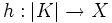Polyhedron

This article defines a property of topological spaces: a property that can be evaluated to true/false for any topological space|View a complete list of properties of topological spaces

Definition

Symbol-free definition

A topological space is termed a polyhedron if there is a homeomorphism to it from the underlying space (viz, geometric realization) of a (finite) simplicial complex. The simplicial complex, along with the homeomorphism, is termed a triangulation of the topological space.

Definition with symbols

A topological space$X$ is termed a polyhedron if there is a (finite) simplicial complex$K$ and a homeomorphism$h:|K| \to X$. The pair$(K,h)$ is termed a triangulation of$X$.